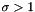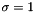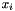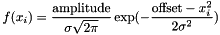Gromacs  2020.1
gmx::GaussianOn1DLattice Class Reference

#include <gromacs/math/gausstransform.h>

## Description

Provide result of Gaussian function evaluation on a one-dimensional lattice.

This class owns the result of the operation and provides a view on it.

The distance between lattice points is one. Unit length is normalized by the lattice spacing, thus spreading width and range are given as multiples of lattice points distances.

This works well as approximation to piece-wise integration of a Gaussian on a lattice, when. The maximal relative error to evaluating erf differences foris 0.01602.

## Public Member Functions

GaussianOn1DLattice (const GaussianOn1DLattice &other)
Copy constructor.

GaussianOn1DLatticeoperator= (const GaussianOn1DLattice &other)
Copy assignment.

GaussianOn1DLattice (GaussianOn1DLattice &&other) noexcept
Move constructor.

GaussianOn1DLatticeoperator= (GaussianOn1DLattice &&other) noexcept
Move assignment.

void spread (double amplitude, real latticeOffset)
Spreads weight onto grid points in one dimension. More...

ArrayRef< const float > view ()

## Constructor & Destructor Documentation

 gmx::GaussianOn1DLattice::GaussianOn1DLattice ( int numGridPointsForSpreadingHalfWidth, real sigma )

Spread weights are distributed over a non-periodic lattice of length 2*numGridPointsForSpreadingHalfWidth+1. The lattice comprises a center point and spreadDistance points to the left and to the right.

Note
There is a maximum spreading width
Parameters
 [in] numGridPointsForSpreadingHalfWidth maximum distance in number of gridpoints from 0 [in] sigma Gaussian width.

## Member Function Documentation

 void gmx::GaussianOn1DLattice::spread ( double amplitude, real latticeOffset )

Spreads weight onto grid points in one dimension.

       .            :            |            :            .
o            o            o            o            o
O---|
latticeOffset


O - atom position o - lattice positions . : | spreading value at grid points.

Note
Highest numerical accuracy is achieved when the spreading with offset to the nearest lattice coordinated < 0.5

Spreading on lattice coordinateParameters
 [in] amplitude of the Gaussian spread. [in] latticeOffset The distance to the nearest grid point in lattice coordinates.

The documentation for this class was generated from the following files: